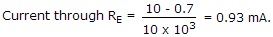# Electronics and Communication Engineering - Analog Electronics

1.

Figure is a 24 Vr stabilized power supply. The zener is 24 V, 600 mW. The minimum zener current is 10 mA. Proper values R and maximum load current are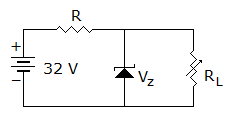A. 320 Ω, 10 mA B. 400 Ω, 15 mA C. 400 Ω, 10 mA D. 320 Ω, 15 mA

Explanation:

Max. zener current =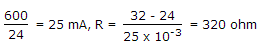.

Maximum load current = 25 - 10 = 15 mA.

2.

The open loop gain of an amplifier is 200. If negative feedback with β = 0.2 is used, the closed loop gain will be

 A. 200 B. 40.12 C. 4.878 D. 2.2

Explanation:

Closed loop gain =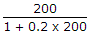.

3.

In a class C power amplifier the input signal has a frequency of 250 kHz. If the collector current pulses are 0.1 μs wide, the duty cycle of current waveform is

 A. 50% B. 25% C. 2.5% D. 0.25%

Explanation: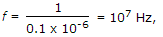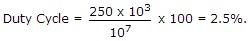4.

In figure as the load resistance is changed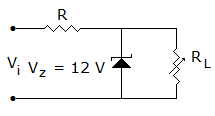A. the zener current remain constant B. the load current remains constant C. the output voltage remains constant D. the output voltage and current through R remain constant

Explanation:

Output voltage = 12 V,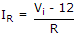.

5.

In figure, voltage across R2 = + 10 V. If VBE = 0.7 V and VE = 0.7 V and RE = 10 kΩ, current through RE is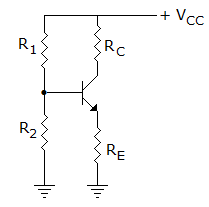A. 1 mA B. 0.93 mA C. 2 mA D. 1.86 mA